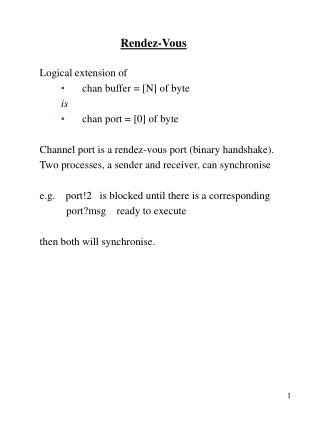Download PresentationRendez-Vous

# Rendez-Vous - PowerPoint PPT PresentationDownload Presentation## Rendez-Vous

- - - - - - - - - - - - - - - - - - - - - - - - - - - E N D - - - - - - - - - - - - - - - - - - - - - - - - - - -
##### Presentation Transcript

1. Rendez-Vous Logical extension of chan buffer = [N] of byte is chan port =  of byte Channel port is a rendez-vous port (binary handshake). Two processes, a sender and receiver, can synchronise e.g. port!2 is blocked until there is a corresponding port?msg ready to execute then both will synchronise.

2. Dijkstra’s Semaphore using Rendez-vous #define p 0 #define q 1 chan sema =  of {bit}; proctype semaphore() { byte count = 1; do :: (count == 1) -> sema!p; count = 0 :: (count == 0) -> sema?v; count = 1 od } proctype user() { do :: sema?p; /* critical section */ sema!v /* non-critical section */ od } init { run semaphore(); run user(); run user(); run user() } 1,2,3,1,2,3,1,2,3, ... 1,2,3,3,2,1,1,2,3,3,2,1, … 1,1,1,1,1,1,1,1,1, ... etc.

3. Synchronous vs Asynchronous Communication #define msgtype … chan name = [x] of {byte,byte} proctype A() { name!msgtype(124); name!msgtype(121) } proctype B() { byte state; name?state } init { atomic {run A(); run B()}} /* created at the same time */ Behaviour x==0 A and B will synch on transfer of 124, then A will block. x == 1 A can send 124, then blocks until B reads it. A can then send 121. Both processes complete, but 121 is still on name. x >= 2 A can complete without B ever starting.

4. An Interesting Way to Compute the Factorial Function Proctype fact(int n; chan p) /* calculate factorial n, communicating result via p */ {chan child =  of {int}; /* for result from fact n-1 */ int result; if :: (n <= 1) -> p!1 :: (n>=2) -> run fact(n-1, child); child?result; p!(n*result) fi } init /* factorial 5 */ { chan result =  of {int}; int answer; run fact(5, result); result?answer; printf(“result is “%d\n”, result) }

5. Assertions Assertions are statements about the program state that can be embedded in the program. assert (condition) E.g. assert (state == 1) assert (x >= y) Extremely useful! For run-time behavioural audit program invariants But be careful, assertions abort the program if the condition evaluates to 0, i.e. it is false.

6. Assertions Common ways to use assertions: idle: assert(arm == up); ….. receiver: assert (full(inchannel)); parcel == 0; ….. sender: assert (empty(outchannel)); parcel == 1; ... inchannel?x; assert x == last_value + 1; …..

7. Factorial Function Proctype fact(int n; chan p) /* calculate factorial n, communicating result via p */ {chan child =  of {int}; /* for result from fact n-1 */ int result; if :: (n <= 1) -> p!1 :: (n>=2) -> assert (empty child); run fact(n-1, child); assert (full(child)); child?result; p!(n*result) fi (assert full(p)) } init /* factorial 5 */ { chan result =  of int; int answer; run fact(5, result); answer?result; assert( result == 120); printf(“result is “%d\n”, result) }